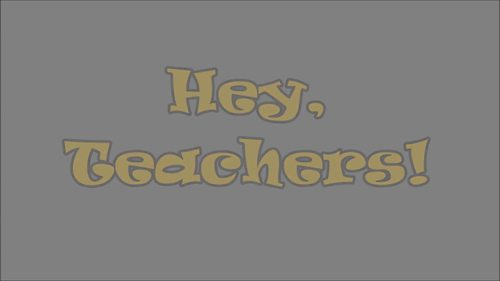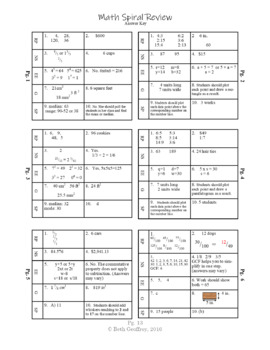# 6th grade Math Spiral Review

Rated 4.9 out of 5, based on 10 reviews
10 Ratings;
6th - 7th
Subjects
Resource Type
Standards
Formats Included
• PDF
Pages
17 pages
Report this resource to TPT
##### Also included in
1. 6th grade math spiral review BUNDLE - Printable PDF worksheets that cover all 5 common core standards: Ratios and Proportions, The Number System, Expressions and Equations, Geometry, &amp; Statistics and Probability. 42 work pages with answer keys full of skill and word problems. Great for centers,
Price \$16.99Original Price \$19.99Save \$3.00
2. This is a great resource to help 6th graders review math skills, and perfect for returning 7th graders! Use these items in centers, as morning work, homework, test prep, and more! Each printable page contains both basic skill practice along with related word problems. The digital task cards (Boom Ca
Price \$19.00Original Price \$23.69Save \$4.69

### Description

6th grade Spiral Math Review - Printable PDF worksheets that cover all 5 common core standards: Ratios and Proportions, The Number System, Expressions and Equations, Geometry, & Statistics and Probability. 12 work pages with answer keys full of skill and word problems. Great for centers, homework, morning work, bell ringers, homeschool, test prep, test review, and extra practice! Makes a great beginning of year assessment for incoming 7th graders!

Each page features 2 problems within a specific standard or focus area: one skill problem and one word problem, for a total of 10 problems per page - plenty for a weekly assignment.

I have designed this review to specifically help with connecting the concrete skill practice to application within word problems. Each word problem on the right is directly related to the skill that is being practiced on the left of each page.

• 12 student work pages – each covering all 5 sixth grade math domains.

• Suggestions and guidance on how to use this resource in the classroom.

Bundle and Save! - This resource is also part of my 6th-Grade Math Spiral Review BUNDLE.

Try my Domain Series Focus Reviews. They pair perfectly with this resource!

Total Pages
17 pages
Included
Teaching Duration
N/A
Report this resource to TPT
Reported resources will be reviewed by our team. Report this resource to let us know if this resource violates TPT’s content guidelines.

### Standards

to see state-specific standards (only available in the US).
Find the area of right triangles, other triangles, special quadrilaterals, and polygons by composing into rectangles or decomposing into triangles and other shapes; apply these techniques in the context of solving real-world and mathematical problems.
Find the volume of a right rectangular prism with fractional edge lengths by packing it with unit cubes of the appropriate unit fraction edge lengths, and show that the volume is the same as would be found by multiplying the edge lengths of the prism. Apply the formulas 𝘝 = 𝘭 𝘸 𝘩 and 𝘝 = 𝘣 𝘩 to find volumes of right rectangular prisms with fractional edge lengths in the context of solving real-world and mathematical problems.
Draw polygons in the coordinate plane given coordinates for the vertices; use coordinates to find the length of a side joining points with the same first coordinate or the same second coordinate. Apply these techniques in the context of solving real-world and mathematical problems.
Represent three-dimensional figures using nets made up of rectangles and triangles, and use the nets to find the surface area of these figures. Apply these techniques in the context of solving real-world and mathematical problems.
Understand that a set of data collected to answer a statistical question has a distribution which can be described by its center, spread, and overall shape.